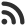Feed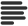Articles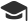Tutorials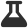CodingLab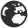CommunityLeaderboardFounder & Programmer at HackersFriend Updated Jan. 8, 2020, 8:12 a.m. ⋅ 93 views

# Sort string of characters C++

We can sort the characters of a string using sort function that comes with C++. We need to pass the start and end position the string like sort(str.begin(), str.end()). This will sort the string the O(n log n) time complexity.

Let's take a look at how it works.

Input

``hackersfriend``

Output

``acdeefhiknrrs``

Implemenation

``````// C++ program to sort a string of characters
#include<bits/stdc++.h>
using namespace std;

int main()
{
string s = "hackersfriend";
// it is inplace so it will modify the original s
sort(s.begin(), s.end());
cout<<s;
return 0;
}
``````
``acdeefhiknrrs ``

Efficient apporach for large inputs

We can imporve this approach by building a hash map of the characters of string, since there are total 256 charcters possible as per ascii value. We can build a hash map of 256 character and then we can store the occurance of each character in our hash map in one iteration of string then, we can print the total elements from our hash map, as many times is their frequncy.

This appraoch would be of O (n) complexity. Let's implement this approach.

``````// C++ program to sort a string of characters
#include<bits/stdc++.h>
using namespace std;

int main()
{

string s = "hackersfriend123";

int hash_map = { 0 };

for(int i = 0; i<s.length(); i++)
{
hash_map[int(s[i])]++;
}

// hash map is created
// lets print all the elements from it
for(int i=0; i<256; i++)
{
for(int j=1; j<=hash_map[i]; j++)
{
if(hash_map[i] != 0)
cout<<char(i);
}
}

return 0;

}``````

``123acdeefhiknrrs ``

 arrow_upward UpvoteComment arrow_downward downvote

Events

Nov. 28, 2018, 5:30 p.m.

Python from zero to hero

place Delhi

Aug. 13, 2018, 5:30 p.m.

Python from zero to hero

place Bangalore ( HackersFriend office BTM Layout)